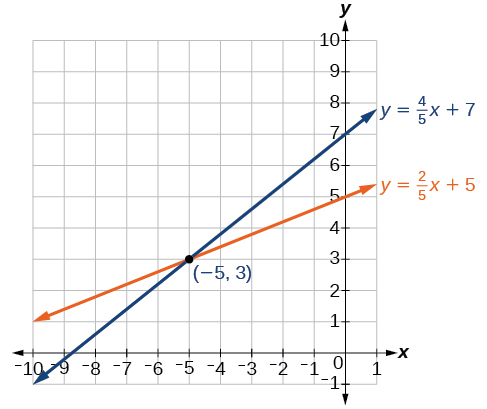# 11.1 Systems of linear equations: two variables  (Page 2/20)

 Page 2 / 20

Another type of system of linear equations is an inconsistent system , which is one in which the equations represent two parallel lines. The lines have the same slope and different y- intercepts. There are no points common to both lines; hence, there is no solution to the system.

## Types of linear systems

There are three types of systems of linear equations in two variables, and three types of solutions.

• An independent system    has exactly one solution pair $\text{\hspace{0.17em}}\left(x,y\right).\text{\hspace{0.17em}}$ The point where the two lines intersect is the only solution.
• An inconsistent system    has no solution. Notice that the two lines are parallel and will never intersect.
• A dependent system    has infinitely many solutions. The lines are coincident. They are the same line, so every coordinate pair on the line is a solution to both equations.

[link] compares graphical representations of each type of system.

Given a system of linear equations and an ordered pair, determine whether the ordered pair is a solution.

1. Substitute the ordered pair into each equation in the system.
2. Determine whether true statements result from the substitution in both equations; if so, the ordered pair is a solution.

## Determining whether an ordered pair is a solution to a system of equations

Determine whether the ordered pair $\text{\hspace{0.17em}}\left(5,1\right)\text{\hspace{0.17em}}$ is a solution to the given system of equations.

$\begin{array}{l}\text{\hspace{0.17em}}x+3y=8\hfill \\ \text{\hspace{0.17em}}2x-9=y\hfill \end{array}$

Substitute the ordered pair $\text{\hspace{0.17em}}\left(5,1\right)\text{\hspace{0.17em}}$ into both equations.

The ordered pair $\text{\hspace{0.17em}}\left(5,1\right)\text{\hspace{0.17em}}$ satisfies both equations, so it is the solution to the system.

Determine whether the ordered pair $\text{\hspace{0.17em}}\left(8,5\right)\text{\hspace{0.17em}}$ is a solution to the following system.

$\begin{array}{c}5x-4y=20\\ \text{\hspace{0.17em}}\text{\hspace{0.17em}}\text{\hspace{0.17em}}2x+1=3y\end{array}$

Not a solution.

## Solving systems of equations by graphing

There are multiple methods of solving systems of linear equations. For a system of linear equations    in two variables, we can determine both the type of system and the solution by graphing the system of equations on the same set of axes.

## Solving a system of equations in two variables by graphing

Solve the following system of equations by graphing. Identify the type of system.

$\begin{array}{c}2x+y=-8\\ \text{\hspace{0.17em}}\text{\hspace{0.17em}}\text{\hspace{0.17em}}x-y=-1\end{array}$

Solve the first equation for $\text{\hspace{0.17em}}y.$

$\begin{array}{c}2x+y=-8\\ \text{\hspace{0.17em}}\text{\hspace{0.17em}}\text{\hspace{0.17em}}\text{\hspace{0.17em}}\text{\hspace{0.17em}}\text{\hspace{0.17em}}\text{\hspace{0.17em}}\text{\hspace{0.17em}}\text{\hspace{0.17em}}\text{\hspace{0.17em}}\text{\hspace{0.17em}}\text{\hspace{0.17em}}\text{\hspace{0.17em}}\text{\hspace{0.17em}}\text{\hspace{0.17em}}\text{\hspace{0.17em}}\text{\hspace{0.17em}}\text{\hspace{0.17em}}\text{\hspace{0.17em}}\text{\hspace{0.17em}}\text{\hspace{0.17em}}\text{\hspace{0.17em}}\text{\hspace{0.17em}}\text{\hspace{0.17em}}\text{\hspace{0.17em}}\text{\hspace{0.17em}}y=-2x-8\end{array}$

Solve the second equation for $\text{\hspace{0.17em}}y.$

$\begin{array}{c}x-y=-1\\ \text{\hspace{0.17em}}\text{\hspace{0.17em}}\text{\hspace{0.17em}}\text{\hspace{0.17em}}\text{\hspace{0.17em}}\text{\hspace{0.17em}}\text{\hspace{0.17em}}\text{\hspace{0.17em}}\text{\hspace{0.17em}}\text{\hspace{0.17em}}\text{\hspace{0.17em}}\text{\hspace{0.17em}}\text{\hspace{0.17em}}\text{\hspace{0.17em}}\text{\hspace{0.17em}}y=x+1\end{array}$

Graph both equations on the same set of axes as in [link] .

The lines appear to intersect at the point $\text{\hspace{0.17em}}\left(-3,-2\right).\text{\hspace{0.17em}}$ We can check to make sure that this is the solution to the system by substituting the ordered pair into both equations.

The solution to the system is the ordered pair $\text{\hspace{0.17em}}\left(-3,-2\right),$ so the system is independent.

Solve the following system of equations by graphing.

The solution to the system is the ordered pair $\text{\hspace{0.17em}}\left(-5,3\right).$Can graphing be used if the system is inconsistent or dependent?

Yes, in both cases we can still graph the system to determine the type of system and solution. If the two lines are parallel, the system has no solution and is inconsistent. If the two lines are identical, the system has infinite solutions and is a dependent system.

Find the possible value of 8.5 using moivre's theorem
which of these functions is not uniformly cintinuous on (0, 1)? sinx
which of these functions is not uniformly continuous on 0,1
solve this equation by completing the square 3x-4x-7=0
X=7
Muustapha
=7
mantu
x=7
mantu
3x-4x-7=0 -x=7 x=-7
Kr
x=-7
mantu
9x-16x-49=0 -7x=49 -x=7 x=7
mantu
what's the formula
Modress
-x=7
Modress
new member
siame
what is trigonometry
deals with circles, angles, and triangles. Usually in the form of Soh cah toa or sine, cosine, and tangent
Thomas
solve for me this equational y=2-x
what are you solving for
Alex
solve x
Rubben
you would move everything to the other side leaving x by itself. subtract 2 and divide -1.
Nikki
then I got x=-2
Rubben
it will b -y+2=x
Alex
goodness. I'm sorry. I will let Alex take the wheel.
Nikki
ouky thanks braa
Rubben
I think he drive me safe
Rubben
how to get 8 trigonometric function of tanA=0.5, given SinA=5/13? Can you help me?m
More example of algebra and trigo
What is Indices
If one side only of a triangle is given is it possible to solve for the unkown two sides?
cool
Rubben
kya
Khushnama
please I need help in maths
Okey tell me, what's your problem is?
Navin
the least possible degree ?
(1+cosA)(1-cosA)=sin^2A
good
Neha
why I'm sending you solved question
Mirza
Teach me abt the echelon method
Khamis
exact value of cos(π/3-π/4)
What is differentiation?ByByByByBy Sam LuongByBy Edward Biton